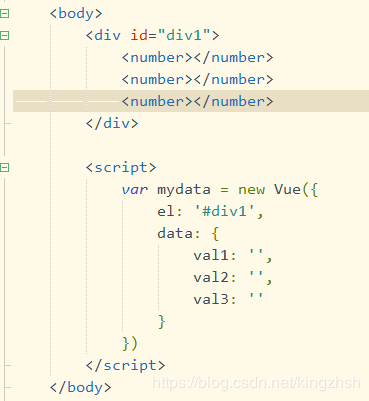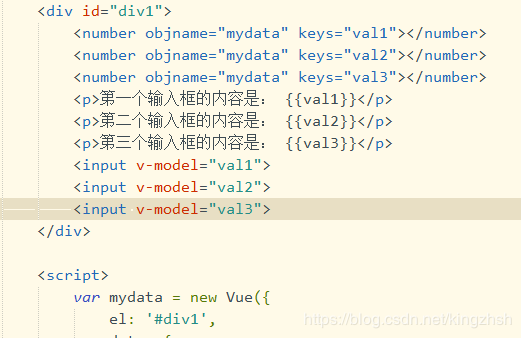# Vue.js实现只能输入数字的输入框_情愿_前端开发者

```<!DOCTYPE html>
<html>
<meta charset="utf-8">
<title>vue</title>
<script src="https://cdn.staticfile.org/vue/2.4.2/vue.min.js"></script>
<body>
<div id="div1">
<input v-model="content">
</div>
<script>
var mydata = new vue({
el: '#div1',
data: {
content: ''
},
watch: {
content: function(val){
this.content = val.replace(/\D/g, '')
}
}
})
</script>
</body>
</html>```

```vue.component('number', {
template: '<input type="text" v-model="con">',
data: function(){
return {
con: ''
}
},
watch: {
con: function(val){
this.con = val.replace(/\D/g, '');
}
}
})``````<!DOCTYPE html>
<html>
<meta charset="utf-8">
<title>vue</title>
<script src="https://cdn.staticfile.org/vue/2.4.2/vue.min.js"></script>
<script src="num.js"></script>
<body>
<div id="div1">
<number objname="mydata" keys="val1"></number>
<number objname="mydata" keys="val2"></number>
<number objname="mydata" keys="val3"></number>
<p>第一个输入框的内容是： {{val1}}</p>
<p>第二个输入框的内容是： {{val2}}</p>
<p>第三个输入框的内容是： {{val3}}</p>
</div>

<script>
var mydata = new Vue({
el: '#div1',
data: {
val1: '',
val2: '',
val3: ''
}
})
</script>
</body>
</html>```

num.js的代码：

```Vue.component('number', {
props: ['objname', 'keys'],
template: '<input type="text" v-model="con">',
data: function(){
return {
con: ''
}
},
watch: {
con: function(val){
this.con = val.replace(/\D/g, '');
eval(this.objname+'[this.keys]=this.con');
}
},
})``````<div id="div1">
<number ref="val1" objname="mydata" keys="val1"></number>
<number ref="val2" objname="mydata" keys="val2"></number>
<number ref="val3" objname="mydata" keys="val3"></number>
<p>第一个输入框的内容是： {{val1}}</p>
<p>第二个输入框的内容是： {{val2}}</p>
<p>第三个输入框的内容是： {{val3}}</p>
<input v-model="val1">
<input v-model="val2">
<input v-model="val3">
</div>```

num.js里面

```Vue.component('number', {
props: ['objname', 'keys'],
template: '<input type="text" v-model="con">',
data: function(){
return {
con: ''
}
},
mounted: function(){
var self = this;
eval(self.objname+'.\$watch(\''+self.keys+'\',function(val){this.\$refs.'+self.keys+'.con=val})');
})
},
watch: {
con: function(val){
this.con = val.replace(/\D/g, '');
eval(this.objname+'[this.keys]=this.con');
}
},
})```

» 本文链接地址：https://www.rokub.com/70878.html
» 您也可以订阅本站：https://www.rokub.com
64K

• 回顶